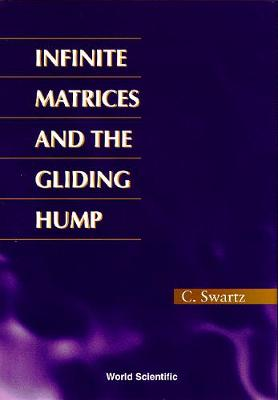•# Infinite Matrices And The Gliding Hump, Matrix Methods In Analysis (Hardback)

(author)
£62.00
Hardback 224 Pages / Published: 22/08/1996
• We can order this

Usually dispatched within 15 working days

These notes present a theorem on infinite matrices with values in a topological group due to P Antosik and J Mikusinski. Using the matrix theorem and classical gliding hump techniques, a number of applications to various topics in functional analysis, measure theory and sequence spaces are given. There are a number of generalizations of the classical Uniform Boundedness Principle given; in particular, using stronger notions of sequential convergence and boundedness due to Antosik and Mikusinski, versions of the Uniform Boundedness Principle and the Banach-Steinhaus Theorem are given which, in contrast to the usual versions, require no completeness or barrelledness assumptions on the domain space. Versions of Nikodym Boundedness and Convergence Theorems of measure theory, the Orlicz-Pettis Theorem on subseries convergence, generalizations of the Schur Lemma on the equivalence of weak and norm convergence in l1 and the Mazur-Orlicz Theorem on the continuity of separately continuous bilinear mappings are also given. Finally, the matrix theorems are also employed to treat a number of topics in sequence spaces.

Publisher: World Scientific Publishing Co Pte Ltd
ISBN: 9789810227364
Number of pages: 224